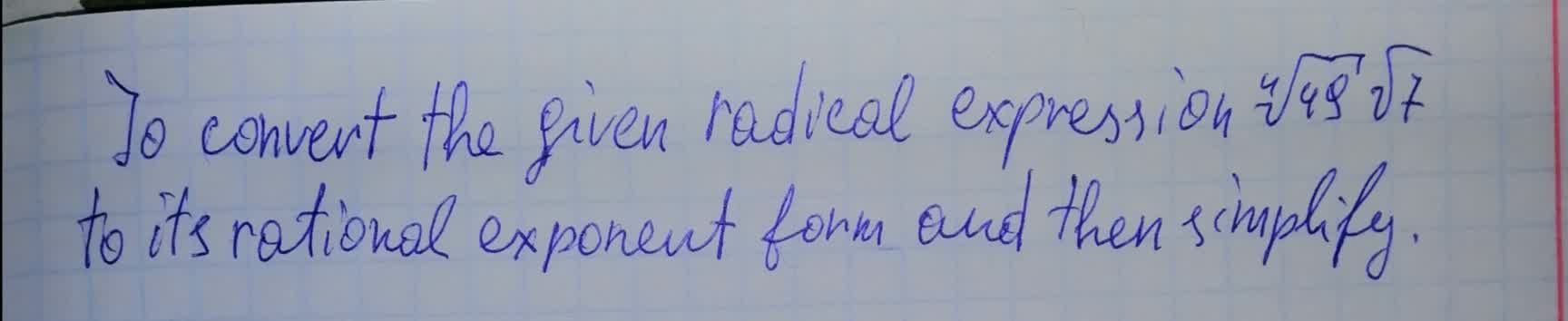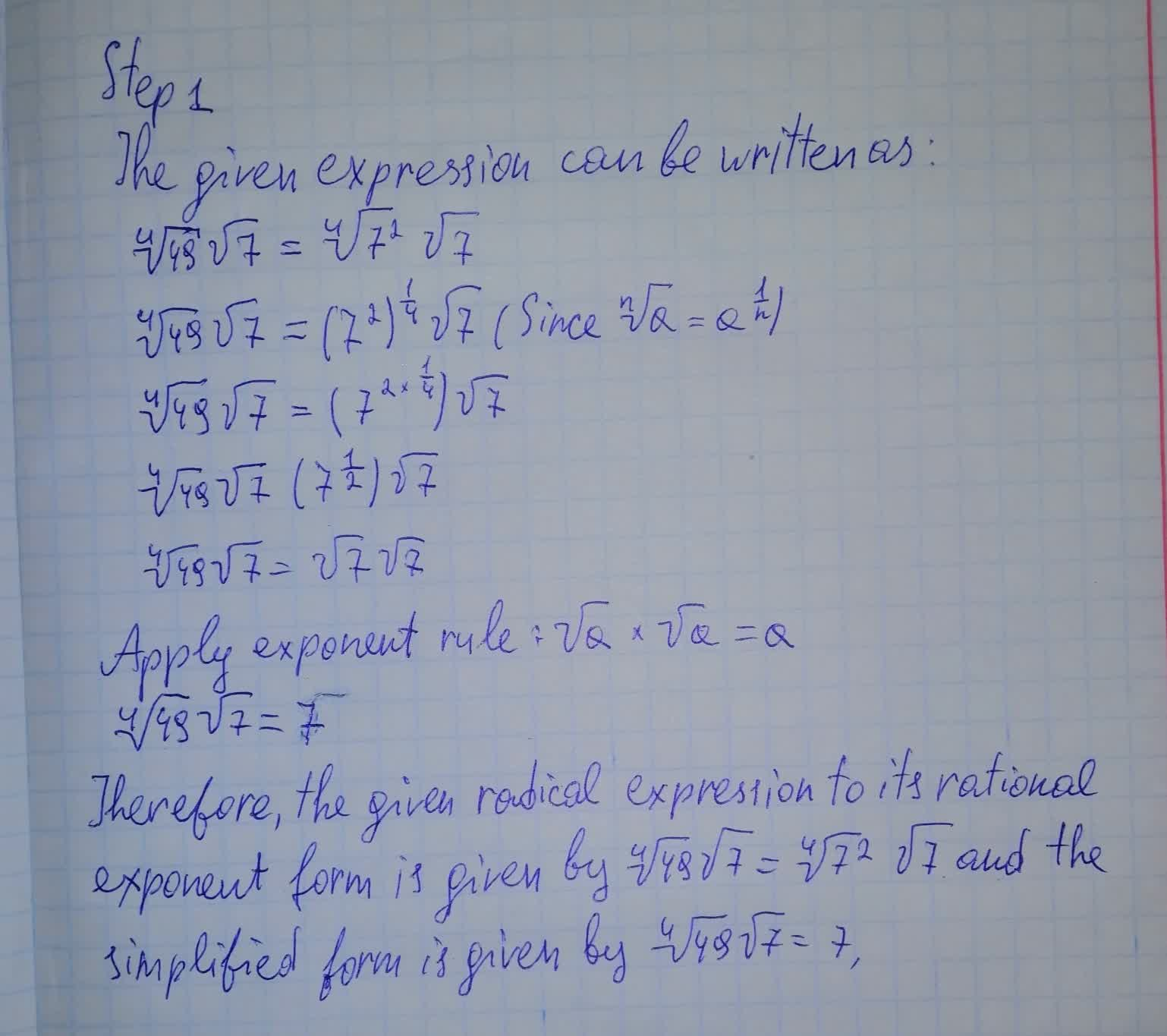Question# To convert the given radical expression \sqrt{49}\sqrt{7} to its rational exponent form and then simplify.

Conic sections
ANSWEREDTo convert the given radical expression $$\displaystyle\sqrt{49}\sqrt{{{7}}}$$ to its rational exponent form and then simplify.